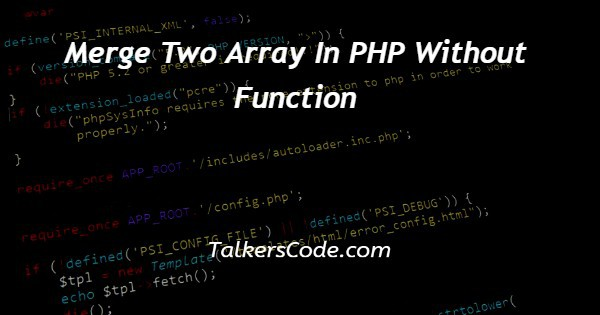# Merge Two Array In PHP Without Function

Last Updated : Jul 1, 2023In this tutorial we will show you the solution of merge two array in PHP without function, in PHP, while creating web pages we face various conditions where we have to merge the elements of arrays.

Merging two or more arrays by using any function makes it complex to understand code for the developer,

On considering that, in this tutorial we’re going to understand the steps and code to merge two arrays in PHP without using any specific function or array_merge function.

## Step By Step Guide On Merge Two Array In PHP Without Function :-

### Merged array use in PHP -

1. We can assign one array to the function and as many as we want simultaneously.
2. If it has same key elements of array, the last one will override the other one.

Now, we’re going to merge two arrays in PHP by referring to an example -

```<?php
\$array_a = ['rohan','raj','rahul'];
\$array_b = ['laxman','lucky','logan'];
\$array_z = array();
for(\$i=0;\$i<count(\$array_a); \$i++ ){
\$array_z[\$i] = \$array_a[\$i];
}
\$count = count(\$array_a);
for(\$i=(\$count),\$j=0;\$i<count(\$array_b)+\$count; \$i++,\$j++){
\$array_z[\$i] = \$array_b[\$j];
}
print_r(\$array_z);
?>```
1. First, we’ve declared two arrays as array_a and array_b then specified their respective values and array_z as array function without any values.
2. Then we used for loop by incrementing the values using i++ in order to print the respective values.
3. Now, we specified array_z as equal to array_a and array_b in the code.
4. Again we used for loop for array_b and then initialized it’s values by making it equal to array_z.
5. At the end, we printed the array_z, which has been already declared and initialized with both the previous arrays earlier.
6. Print_r() function will print all the elements of both the arrays.

### Other Method -

1. We can also merge two arrays, by using array_merge() function.
2. array_merge() function also used to merge two arrays without declaring it equal to another very easily.
```       <?php
\$name = array('rahul','rohan','raj');
\$age = array(12,23,43);
\$mergedarray = array_merge(\$name,\$age);
print_r(\$mergedarray);
?>```
1. Here, we used array_merged function to merge two arrays(name and age) together without any initialization.
2. We directly merged both of them by using array_merge function.

## Conclusion :-

In this tutorial, we’ve understood how we can merge two arrays directly or by using function in PHP. I hope this tutorial on merge two array in PHP without function helps you.